Open in App
Not now

# Deadlock Avoidance in Distributed System

• Last Updated : 26 May, 2022

Deadlocks are the fundamental problems in distributed systems. A Deadlock is a situation where a set of processes are blocked as each process in a Distributed system is holding some resources and that acquired resources are needed by some other processes. In this situation, a cycle arrives at a deadlock.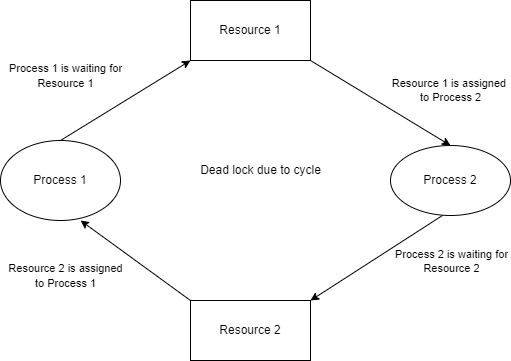Conditions of deadlock: A process in distributed systems uses different resources in the following way.

• Request
• Use
• Releases

That means, a process requests the resource and uses it in its execution and after execution, it releases the resource.

There are four methods for handling Deadlock:

In Deadlock Avoidance, the system will be checked if it is in a safe state or an unsafe state. Safe state is ensured when the request for the resource by the process is permitted when there is no deadlock found in the system. If there is deadlock found then the system will be in an unsafe state.

To avoid deadlocks the process should inform the system that how many resources that a process should request for its execution. To make that happen we use Algorithm.

Step 1: Work and finish the 2 vectors of size m & n. Initialize work with available and finish[i] = false

for i=1 to n , m=>#resources and n=>#processes.

Step 2: Find an i such that both (i) finish[i] = false (ii) need(i) <= work , if no such i exists then go to Step 4.

Step 3: Work = work + allocation

finish[i] = true

go to Step 2.

Step 4: if finish[i] = true for all i then the system is in a safe state.

Suppose that there are m = 4 (A, B, C, D) resources and n=5 (P0, P1, P2, P3, P4) processes. Build a safe sequence to keep the system in a safe state.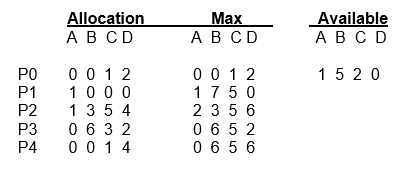Here,

```n => #processes = 5 = <P0, P1, P2, P3, P4>
m => #resources = 4 = (A, B, C, D)```

Available: It defines how many instances that are available for a particular resource. In the above example A – 1, B – 5, C – 2, D – 0 resources available.

`Size = Available [m] => 1 D array.`

Allocation: It defines the number of instances that are allocated for all the resources of a particular process. In the above example for Process P0 we are having A – 0, B – 0, C – 1, D – 2 resources allocated.

` Size = Allocation [n x m] => 2 D array.`

Max: It defines the maximum number of instances that are available for all the resources of a particular process. In the above example for Process P0, we are having a maximum of A – 0, B – 0, C – 1, and D – 2 resources available.

`Size = Max [n x m] => 2 D array.`

Need: It tells how many instances will be required more for a resource of a process.

`Size = Need [n x m] => 2 D array.`

To check for a safe sequence first we need to calculate the Need matrix.

`Need(i) = Max(i) - Allocation(i)`

For example, if we take P0 then the need would be:

```A = (0-0)
B = (0-0)
C = (1-1)
D = (2-2)
similarly for all processes.```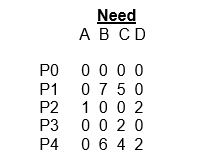After calculating the need matrix now start according to the algorithm.

Step 1: Initialize work and finish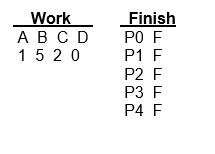Step 2: Now take Process P0 and according to step-2 in algorithm we need to find an i such that both conditions

```(i) finish[i] = false
(ii) need(i) <= work should be satisfied.
we found for P0, finish[i] = false and need(i) <= work ```

such that 0<=1 -> True, 0<=5 -> True, 0<=2 -> True, 0<=0 -> True. So both conditions are satisfied.

Now according to step-3 in algorithm execute (work = work + allocation) & finish[i] = true and go to step-2 in algorithm again.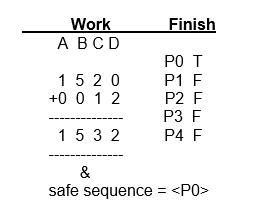Step 3: Now take Process P1, we found finish [i] = false but while coming to need(i) <= work, the condition became false

such that 0<=1 -> True, 7<=5 -> False, 5<=3 -> False, 0<=2 -> True. Condition failed, so skip Process P1 and go to Process P2.

Step 4: For Process P2, we found both the conditions are satisfied. So calculate according to step-3 in the algorithm.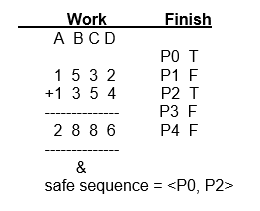Step 5: For Process P3, we found both the conditions are satisfied. So calculate according to step 3 in the algorithm.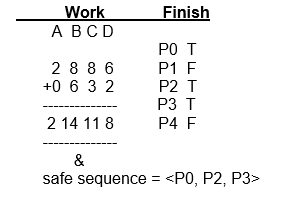Step 6: For Process P4, we found both the conditions are satisfied. So calculate according to step 3 in the algorithm.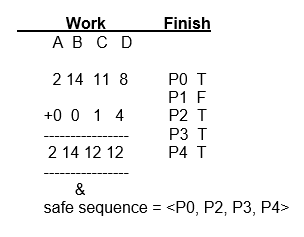Step 7: Now we are remaining with the Process P1. So, if we check for Process P1, we found both the conditions are satisfied. So calculate according to step 3 in the algorithm.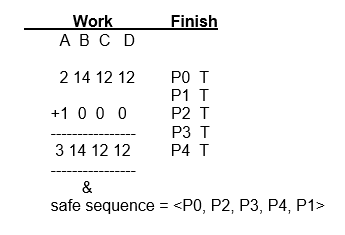Step 8: So when we go to step 2 in the algorithm again we can’t find any ‘i’ for both the conditions as all processes are completed. So now go to step 4 in the algorithm. we find all finish [i] = true. So according to the algorithm, the system is in a safe state.

Finally, the safe sequence for the above example would <P0, P2, P3, P4, P1>.

Cross-checking: We can cross-check the solution by adding the Allocation matrix values for each resource for all the processes with the Available matrix for each resource then it would be the final Work matrix values for each resource.

In the above example if we add

```=> Allocation + Available for resource 'A'
for all processes = 0 + 1 + 1 + 0 + 0 + 1 = 3.
=> Allocation + Available for resource 'B'
for all processes = 0 + 0 + 3 + 6 + 0 + 5 = 14.
=> Allocation + Available for resource 'C'
for all processes = 1 + 0 + 5 + 3 + 1 + 2 = 12.
=> Allocation + Available for resource 'D'
for all processes = 2 + 0 + 4 + 2 + 4 + 0 = 12.```

Here, we can clearly observe the values generated after adding Allocation & Available matrix values are equal to the final. Work matrix values. So the system is in a safe state.